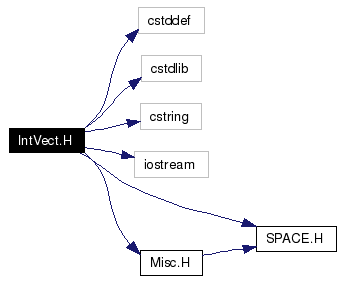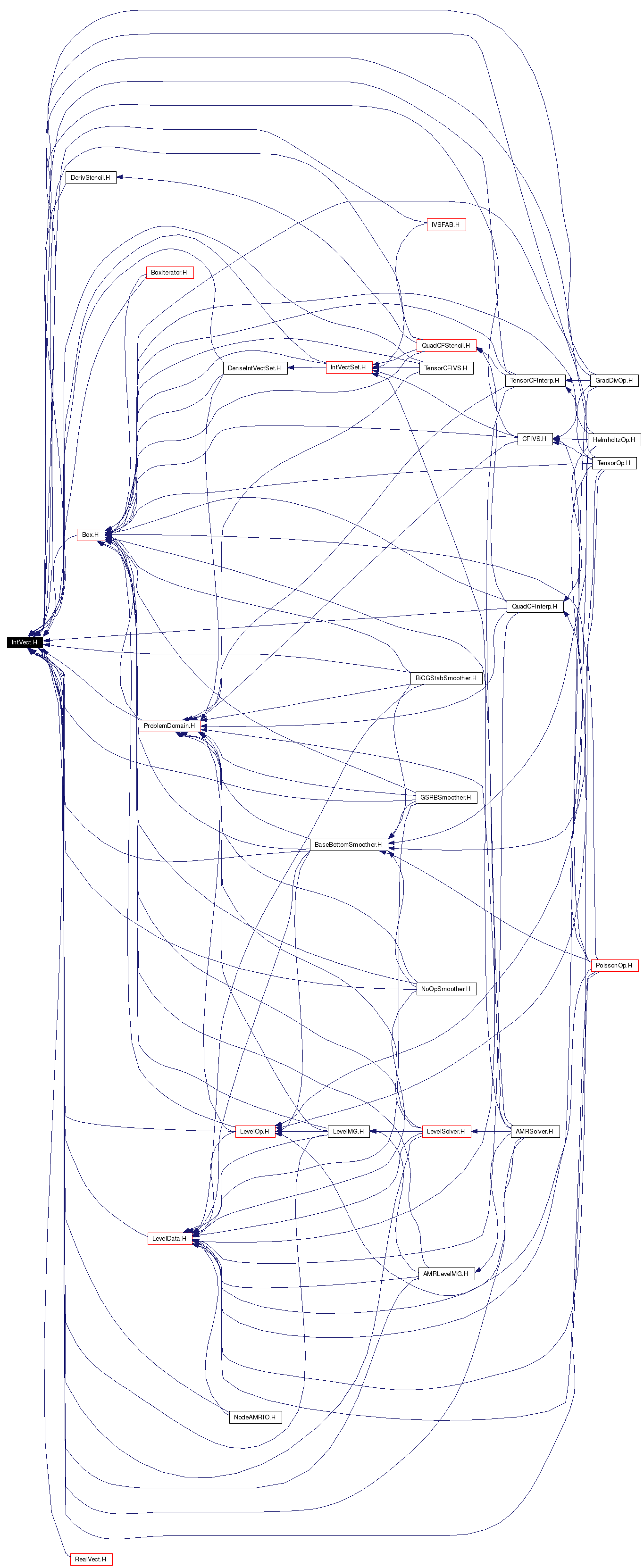# IntVect.H File Reference

`#include <cstddef>`
`#include <cstdlib>`
`#include <cstring>`
`#include <iostream>`
`#include "SPACE.H"`
`#include "Misc.H"`

Include dependency graph for IntVect.H:This graph shows which files directly or indirectly include this file:Go to the source code of this file.

## Defines

#define LLT0   (vect < s)
#define LLT1   ((vect == s) && (vect < s))
#define LLT2   ((vect == s) && (vect < s))
#define LGT0   (vect > s)
#define LGT1   ((vect == s) && (vect > s))
#define LGT2   ((vect == s) && (vect > s))

## Functions

static int s_dummyForIntVectH (IntVect::InitStatics())
IntVect operator+ (int s, const IntVect &p)
IntVect operator- (int s, const IntVect &p)
IntVect operator * (int s, const IntVect &p)
IntVect scale (const IntVect &p, int s)
IntVect diagShift (const IntVect &p, int s)
IntVect min (const IntVect &p1, const IntVect &p2)
IntVect max (const IntVect &p1, const IntVect &p2)

## Define Documentation

 #define LGT0   (vect > s)

 #define LGT1   ((vect == s) && (vect > s))

 #define LGT2   ((vect == s) && (vect > s))

 #define LLT0   (vect < s)

 #define LLT1   ((vect == s) && (vect < s))

 #define LLT2   ((vect == s) && (vect < s))

## Function Documentation

 IntVect diagShift ( const IntVect & p, int s ) ` [inline]`
 Returns IntVect obtained by adding a scalar to each of the components of the given IntVect.

 IntVect max ( const IntVect & p1, const IntVect & p2 ) ` [inline]`
 Returns the IntVect that is the component-wise maximum of two argument IntVects.

 IntVect min ( const IntVect & p1, const IntVect & p2 ) ` [inline]`
 Returns the IntVect that is the component-wise minimum of two argument IntVects.

 IntVect operator * ( int s, const IntVect & p ) ` [inline]`
 Returns an IntVect that is an IntVect p with each component multiplied by a scalar s.

 IntVect operator+ ( int s, const IntVect & p ) ` [inline]`
 Returns an IntVect that is an IntVect p with a scalar s added to each component.

 IntVect operator- ( int s, const IntVect & p ) ` [inline]`
 Returns s - p.

 static int s_dummyForIntVectH ( IntVect:: InitStatics() ) ` [static]`

 IntVect scale ( const IntVect & p, int s ) ` [inline]`
 Returns an IntVect obtained by multiplying each of the components of the given IntVect by a scalar.

Generated on Wed Oct 5 13:55:41 2005 for Chombo&AMRSelfGravity by1.4.1